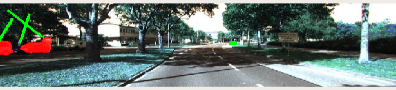# How to create Axis-aligned bounding boxes using Flatten()

I am trying to get axis-aligned bounding box where the original algorithm used rotated bounding boxes. corresponding lines are --

if len(contours) != 0 and np.max(cnt_area) > 100:
contour = contours[np.argmax(cnt_area)]  # use max area polygon
polygon = contour.reshape(-1, 2)
pbox = cv2.boundingRect(polygon)  # Min Max Rectangle
#pbox = cv2.boxPoints(cv2.minAreaRect(polygon))
box_in_img = pbox
state['ploygon'] = rbox_in_img if mask_enable else []

location = state['ploygon'].flatten()


But getting the following error: tuple object doesnot have flatten. but flatten() previously worked with rotated bounding boxes. If I remove flatten to execute the code, the error is gone but I am getting wrong bounding boxes like this--Please give some ideas to solve this issue.

edit retag close merge delete

state['ploygon'] = rbox_in_img if mask_enable else []
• type(state['ploygon']) (probably not a numpy array, so flatten() cannot be applied)
• is ploygon the correct key ? (looks like a typo)